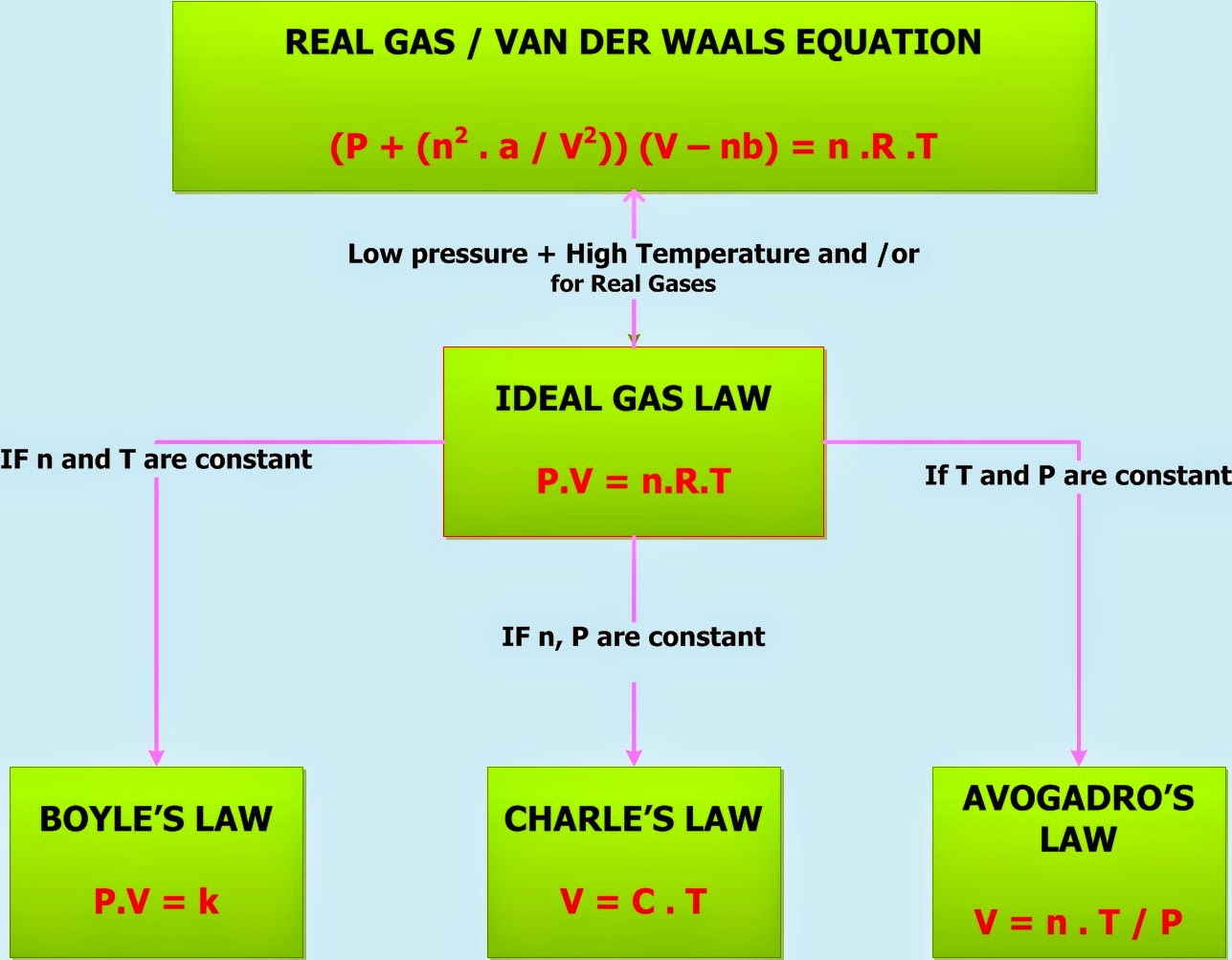Gas Laws – Ideal Gas Law | Chemistry Net

# Gas Laws – Ideal Gas Law

Gas Laws - Ideal Gas Law

# Gas Laws – Ideal Gases

### The Main Gas Laws

The three main gas laws are stated below:

Boyle’s law: The volume of a fixed amount of gas maintained at a constant temperature is inversely proportional to the gas pressure:

P ∝ 1/V  or  P = k/V  or P*V = k   (moles n and temperature T constant)  (1)

Equation (1) shows that the product of the pressure and volume of a fixed amount of gas at a constant temperature T is a constant k.

Charles’ law: The volume of a fixed amount of gas at constant pressure is directly proportional to the temperature T (Kelvin)

V ∝ T  or  V = c * T  (where c, pressure P and moles n constant)     (2)

Avogadro’s law: Equal volumes of different gases compared at the same temperature and pressure contain equal numbers of molecules.

V ∝ n  (P and T constant)        (3)

By combining (1), (2) and (3) above into one proportionality:

V ∝ n*T/P     (4)

Proportionality (4) can be replaced by an equality if a proportionality constant R would be included:

V = R*n*T/P   or    P*V = n*R*T     (5)

This proportionality constant is known as the gas constant R.

Any gas that obeys (1), (2) and (3) will also obey equation (5) which is called the ideal gas equation (Fig. I1) . All gases that obey this equation are called ideal gases.

Under suitable conditions some real gases do approach the behavior of ideal gases and make equation (5) very useful.##### Fig. I1: Interrelationship of the gas laws. Any gas that obeys (1), (2) and (3) will also obey equation (5) which is called the ideal gas equation

The ideal gas equation can be used to establish molecular weights of gases. For this purpose it is helpful to alter the equation slightly by substituting where n (moles of gas) with its equivalent m/MW (where m is the mass of gas and MW its molecular weight) to get the following equation:

P*V = (m/MW)*R*T  (5a)

A solved example regarding the determination of the molecular weight of an ideal gas is presented in the following video:

#### Other Gas Laws

Some other gas laws of note are Raoult’s law, the law of Gaseous Diffusion, Graham’s law and Gay Lussac’s law.

Raoult’s law states: i) the partial pressure of a solute is proportional to the mole fraction of the solute in the solution and ii) the vapor pressure of a solution is directly proportional to the mole fraction of solvent present.

Psoln = xsolvent * Posolvent        (6)

Where Psoln the observed vapor pressure of the solution

Posolvent the vapor pressure of the pure solvent

xsolvent is the mole fraction of the solvent in the solution

From equation (6) can be derived that for a solution that contains half solute molecules and half solvent molecules – xsolvent is 0.5 – the vapor pressure of the solution would be half of the vapor pressure of the solvent.

The effect of the solute on the vapor pressure of a solution gives us a convenient way to “count” molecules and thus provides a means for experimentally determining molar masses. Suppose a certain mass of a compound is dissolved in a solvent, and the vapor pressure of the resulting solution is measured. Using Raoult’s law, we can determine the number of moles of solute present. Since the mass of this number of moles is known, we can calculate the molar mass.

Relevant Posts

References

David W. Oxtoby, H.P. Gillis, Alan Campion, “Principles of Modern Chemistry”, Sixth Edition, Thomson Brooks/Cole, 2008

Ralph H. Petrucci, “General Chemistry”, 3rd Edition, Macmillan Publishing Co., 1982

Key Terms

gas ideal gas law, P.V = nRT, the gas laws, Boyle's gas law,Raoult's law, Graham's law, ideal gas law constant, gas law practice problems, Avogadro's, Graham's, Gay Lussac's, gas constant R, chemistry net, ideal gas law equation